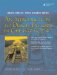# Conversions

A constructor that can be called with a single argument (of a different type) is a conversion constructor because it defines a conversion from the argument type to the constructor's class. See Example 2.16.

Example 2.16. src/ctor/conversion/fraction.cpp

 ```class Fraction { public: Fraction(int n, int d = 1) <-- 1 : m_Numerator(n), m_Denominator(d) {} private: int m_Numerator, m_Denominator; }; int main() { Fraction frac(8); <-- 2 Fraction frac2 = 5; <-- 3 frac = 9; <-- 4 frac = (Fraction) 7; <-- 5 frac = Fraction(6); <-- 6 frac = static_cast(6); <-- 7 return 0; } ```   (1)default argument (2)conversion constructor call (3)copy init (calls conversion ctor too) (4)conversion followed by assignment (5)C-style typecast (deprecated) (6)explicit temporary, also a C++ typecast (7)preferred ANSI style typecast

In Example 2.16, the Fraction variable frac is initialized with a single int. The matching constructor is the two argument version, but the second parameter (denominator) defaults to 1. Effectively, this converts the integer 8 to the fraction 8/1.

The conversion constructor for ClassA is automatically called when an object of that class is required and when such an object can be created by that constructor from the value of TypeB that was supplied as an initializer or assigned value.

For example, if frac is of type Fraction as defined above, then we can write the statement

```frac = 19;
```

Since 19 is not a Fraction object, the compiler checks to see whether it can be converted to a Fraction object. Since we have a conversion constructor, this is indeed possible.

So under the covers, the statement f = 19 makes two "implicit" calls to Fraction member functions:

1. Fraction::Fraction(19); to convert from int to Fraction.
2. Fraction::operator=() to perform the assignment.

This causes a temporary Fraction object on the stack to be created, which is then popped off when the statement is finished executing.

Since we have not defined Fraction::operator=(), the compiler uses a default assignment operator that it supplied.

```Fraction& operator=(const Fraction& fobj);
```

This operator performs a memberwise assignment, from each data member of fobj to the corresponding member of the host object.

Ordinarily, any constructor that can be called with a single argument of different type is a conversion constructor that has the implicit mechanisms discussed here. If the implicit mechanisms are not appropriate for some reason, it is possible to suppress them. The keyword explicit prevents implicit mechanisms from using that constructor.

### const Member FunctionsAn Introduction to Design Patterns in C++ with Qt 4
ISBN: 0131879057
EAN: 2147483647
Year: 2004
Pages: 268